# pcbStack

Single-feed or multifeed PCB antenna

## Description

The `pcbStack` object is a single-feed or multi-feed printed circuit board (PCB) antenna. The `pcbStack` object can be used

• To create single-layer, multilayer metal, or metal-dielectric substrate antennas

• To create an arbitrary number of feeds and vias in an antenna

• To create a PCB antenna with Antenna Toolbox™ antenna catalog elements

• To convert antenna array elements to PCB stack

Note

To generate a Gerber file, a substrate layer is required. Use the `Substrate` property to create this layer in the PCB antenna. For more information, see Stack Conversion

## Creation

### Syntax

``pcbant = pcbStack``
``pcbant = pcbStack(Name,Value)``
``pcbant = pcbStack(ant)``

### Description

``` `pcbant = pcbStack` creates an air-filled single-feed PCB with two metal layers.```

example

``` `pcbant = pcbStack(Name,Value)` sets Properties using name-value pairs. For example, `pcbStack('FeedDiameter', 2.000e-04) ` creates a PCB antenna with a feed diameter of 2.000e-04 meters. You can specify multiple name-value pairs. Enclose each property name in quotes creates a PCB antenna, with additional properties specified by one or more name-value pair arguments. Properties not specified retain their default values.```

example

``` `pcbant = pcbStack(ant)` converts any 2-D or 2.5-D antenna from the antenna catalog into a PCB antenna for further modeling and analysis. You can also use antenna array objects from the antenna array catalog elements them convert it into PCB antennas.```

## Properties

expand all

Name of PCB antenna, specified a character vector.

Example: `'Name','PCBPatch'`

Data Types: `char` | `string`

Revision details of PCB antenna design, specified as a character vector.

Example: `'Revision','2.0'`

Data Types: `char` | `string`

Shape of PC board, specified as an object. The shape can be a rectangle or a polygon.

Example: `'BoardShape',antenna.Polygon`

Thickness of the PC board, specified as a positive scalar. The value of this property is the sum of the thicknesses of all the dielectric layers that lie below the top metal layer. The object treats dielectric layers that are above the top metal layer as coating. Set this property before you set the `Layers` property. For more information on `BoardThickness`, see Board Thickness versus Dielectric Thickness in PCB.

Example: `'BoardThickness',0.02000`

Data Types: `double`

Metal and dielectric layers, specified a cell array of metal layer shapes and dielectric. You can specify one metal shape or one dielectric per layer starting with the top layer and proceeding down.

Data Types: `cell`

Feed locations for PCB antenna in Cartesian coordinates, specified as N -by-3 or N-by-4 array. You can place feed inside the board or at the edge of the board. The arrays translate to the following:

• N -by-3 – [x, y, Layer]

• N-by-4 – [x, y, SigLayer, GndLayer]

Antenna Toolbox uses Delta-Gap source feed model to excite the antenna structure. For more information, see Feed Model

Example: `'FeedLocations',[-0.0187 0 1 2]`

Data Types: `double`

Center pin diameter of feed connector, specified as a positive scalar in meters.

Example: `'FeedDiameter',2.000e-04`

Data Types: `double`

Electrical short locations for antenna in Cartesian coordinates, specified as a real vector of size M-by-4 array. The arrays translate to the following:

• M-by-4 – [x, y, SigLayer, GndLayer]

Example: `'ViaLocations',[0 -0.025 1 2]`

Data Types: `double`

Electrical shorting pin diameter between metal layers, specified as a positive scalar in meters for a single pin or a positive vector in meters for multiple pins. Number of values specified in this property must match the number of pins.

Example: `'ViaDiameter',1.0e-3`

Data Types: `double`

Magnitude voltage applied at the feeds, specified as a positive scalar in volts.

Example: `'FeedVoltage',2`

Data Types: `double`

Model for approximating feed and via, specified as one of the following:

• `'strip'` – A rectangular strip approximation to the feed or via cylinder. This approximation is the simplest and results in a small mesh.

• `'square'` – A 4-sided polyhedron approximation to the feed or via cylinder.

• `'hexagon'` – A 6-sided polyhedron approximation to the feed or via cylinder.

• `'octagon'` – A 8-sided polyhedron approximation to the feed or via cylinder.

Example: `'FeedViaModel','octagon'`

Data Types: `char` | `string`

Excitation phase at each feed, specified as a real vector in degrees.

Example: `'FeedPhase',2`

Data Types: `double`

Type of the metal used as a conductor, specified as a metal material object. You can choose any metal from the `MetalCatalog` or specify a metal of your choice. For more information, see `metal`. For more information on metal conductor meshing, see Meshing.

Example: ```m = metal('Copper'); 'Conductor',m```

Example: ```m = metal('Copper'); ant.Conductor = m```

Lumped elements added to the antenna feed, specified as a lumped element object handle. For more information, see `lumpedElement`.

Example: `'Load',lumpedelement`. `lumpedelement` is the object handle for the load created using `lumpedElement`.

Example: ```pcbant.Load = lumpedElement('Impedance',75)```

Tilt angle of the antenna, specified as a scalar or vector with each element unit in degrees. For more information, see Rotate Antennas and Arrays.

Example: `Tilt=90`

Example: `Tilt=[90 90]`,`TiltAxis=[0 1 0;0 1 1]` tilts the antenna at 90 degrees about the two axes defined by the vectors.

Note

The `wireStack` antenna object only accepts the dot method to change its properties.

Data Types: `double`

Tilt axis of the antenna, specified as:

• Three-element vector of Cartesian coordinates in meters. In this case, each coordinate in the vector starts at the origin and lies along the specified points on the X-, Y-, and Z-axes.

• Two points in space, each specified as three-element vectors of Cartesian coordinates. In this case, the antenna rotates around the line joining the two points in space.

• A string input describing simple rotations around one of the principal axes, 'X', 'Y', or 'Z'.

Example: `TiltAxis=[0 1 0]`

Example: `TiltAxis=[0 0 0;0 1 0]`

Example: `TiltAxis = 'Z'`

Note

The `wireStack` antenna object only accepts the dot method to change its properties.

Data Types: `double`

## Object Functions

 `show` Display antenna, array structures or shapes `array` Create array of PCB stack objects `axialRatio` Axial ratio of antenna `beamwidth` Beamwidth of antenna `charge` Charge distribution on antenna or array surface `current` Current distribution on antenna or array surface `efficiency` Radiation efficiency of antenna `EHfields` Electric and magnetic fields of antennas; Embedded electric and magnetic fields of antenna element in arrays `impedance` Input impedance of antenna; scan impedance of array `info` Display information about antenna or array `layout` Display array or PCB stack layout `mesh` Mesh properties of metal, dielectric antenna, or array structure `meshconfig` Change mesh mode of antenna structure `pattern` Radiation pattern and phase of antenna or array; Embedded pattern of antenna element in array `patternAzimuth` Azimuth pattern of antenna or array `patternElevation` Elevation pattern of antenna or array `returnLoss` Return loss of antenna; scan return loss of array `sparameters` Calculate S-parameter for antenna and antenna array objects `vswr` Voltage standing wave ratio of antenna `plot` Plot boundary of shape

## Examples

collapse all

Setup parameters.

```vp = physconst('lightspeed'); f = 850e6; lambda = vp./f;```

```L = 0.15; W = 1.5*L; stripL = L; gapx = .015; gapy = .01; r1 = antenna.Rectangle('Center',[0,0],'Length',L,'Width',W,'Center',[lambda*0.35,0]); r2 = antenna.Rectangle('Center',[0,0],'Length',L,'Width',W,'Center',[-lambda*0.35,0]); r3 = antenna.Rectangle('Length',0.5*lambda,'Width',0.02*lambda,'NumPoints',2); s = r1 + r2 + r3; figure show(s)```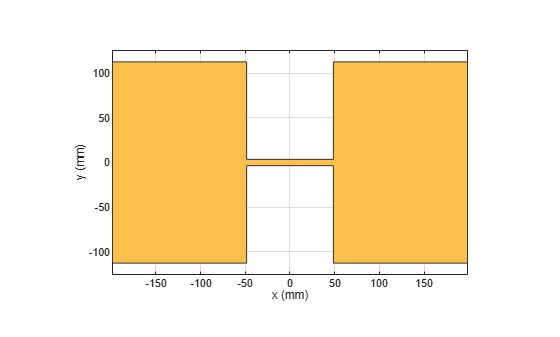Assign the radiator shape to pcbStack and make the changes to the board shape and feed diameter properties.

```boardShape = antenna.Rectangle('Length',0.6,'Width',0.3); p = pcbStack; p.BoardShape = boardShape; p.Layers = {s}; p.FeedDiameter = .02*lambda/2; p.FeedLocations = [0 0 1]; figure show(p)```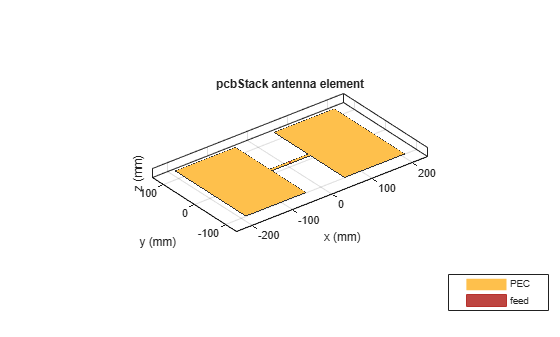Analyze the impedance of the antenna. Effect of the end-loading should result in the series resonance to be pushed lower in the band.

```figure impedance(p,linspace(200e6,1e9,51))```Create a pcb stack antenna with 2 mm dielectric thickness at the radiator and air below it. Display the structure.

```p = pcbStack; d1 = dielectric('FR4'); d1.Thickness = 2e-3; d2 = dielectric('Air'); d2.Thickness = 8e-3; p.Layers = {p.Layers{1},d1,d2,p.Layers{2}}; p.FeedLocations(3:4) = [1 4]; show(p)```Create a PCB stack antenna from reflector backed bowtie.

```b = design(bowtieRounded,1e9); b.Tilt = 90```
```b = bowtieRounded with properties: Length: 0.0959 FlareAngle: 90 Conductor: [1x1 metal] Tilt: 90 TiltAxis: [1 0 0] Load: [1x1 lumpedElement] ```
```b.TiltAxis = [0 1 0]; r = reflector('Exciter',b); p = pcbStack(r);```

Plot the directivity pattern of the antenna at 1 GHz.

`pattern(p,1e9);`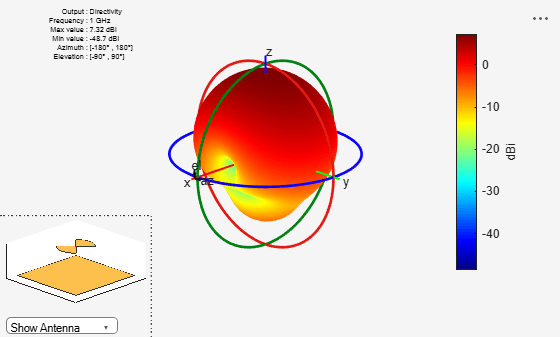Create a coplanar inverted F antenna.

```fco = invertedFcoplanar('Height',14e-3,'GroundPlaneLength', 100e-3, ... 'GroundPlaneWidth', 100e-3);```

Use this antenna to create a `pcbStack` object.

`p = pcbStack(fco);`

Create a circular microstrip patch.

```p = patchMicrostripCircular; d = dielectric; d.EpsilonR = 4.4; p.Radius = .0256; p.Height = 1.6e-3; p.Substrate = d; p.GroundPlaneLength = 3*.0256; p.GroundPlaneWidth = 3*.0256; p.FeedOffset = [.0116 0]; ```

Create a PCB circular microstrip patch using `pcbStack`.

```pb = pcbStack(p); pb.FeedDiameter = 1.27e-3; pb.ViaLocations = [0 pb.FeedLocations(1)/1.1 1 3]; pb.ViaDiameter = pb.FeedDiameter; figure show(pb) ``````C = SMA_Jack_Cinch; O = PCBServices.MayhewWriter; O.DefaultViaDiam = pb.ViaDiameter; O.Filename = 'Microstrip circular patch-9a'; Am = PCBWriter(pb,O,C); gerberWrite(Am) ```

Images using Mayhew Labs 3-D Viewer.Create a coplanar inverted-F antenna.

```fco = invertedFcoplanar('Height',14e-3,'GroundPlaneLength', 100e-3, ... 'GroundPlaneWidth', 100e-3);```

Create a linear array with inverted-F antenna as its elements.

```la = linearArray; la.Element = fco; la.NumElements = 4;```

Use this antenna array to create the PCB antenna.

`p = pcbStack(la);`

Create a `dipole` antenna object and `linearArray` antenna array object. In the` linearArray` antenna object, leave the` Element` property set to its default value of dipole. Set the `ElementSpacing` property to 4."

```d1 = dipole; d2 = linearArray('ElementSpacing', 4);```

To set the Z-coordinate of `pcbStack` antenna object to zero, rotate the dipole and linear dipole array around 90 degrees using the `Tilt `property. Then set the `TiltAxis` property to [ 0 -1 0 ] for dipole and linear dipole array antennas.

```d1.Tilt = 90; d2.Element.Tilt = 90; d1.TiltAxis = [0 -1 0]; d2.Element.TiltAxis = [0 -1 0];```

Create and view PCB stack antenna created using the `dipole` antenna object.

```p1 = pcbStack(d1); show(p1)```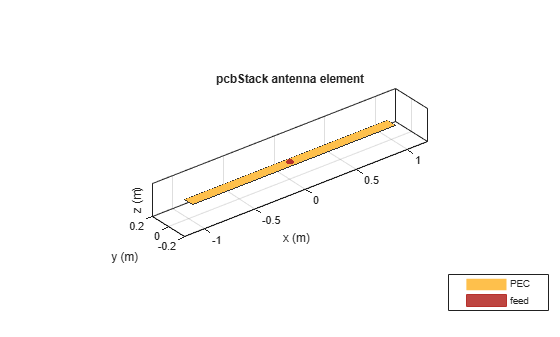Create and view PCB stack antenna using the `linearArray` antenna array object.

```p2 = pcbStack(d2); show(p2)```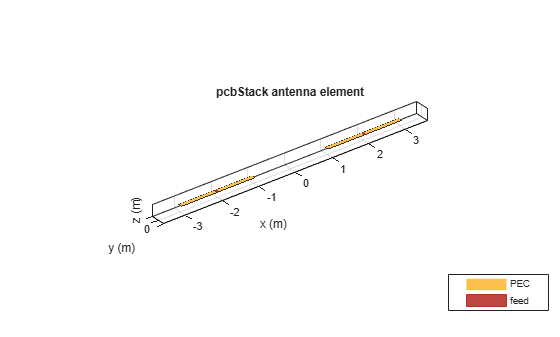Create a circular microstrip patch antenna.

```ant = design(patchMicrostripCircular,3e9); ant.Substrate = dielectric( 'FR4' ); show(ant)```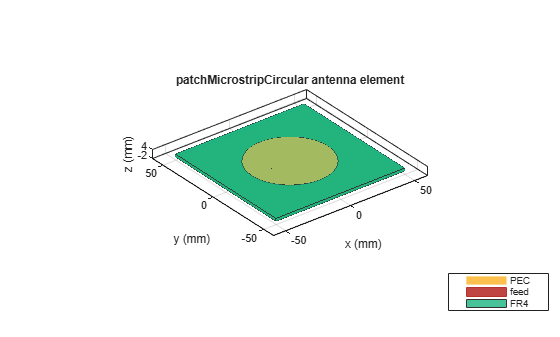```c = antenna.Circle; show(c)```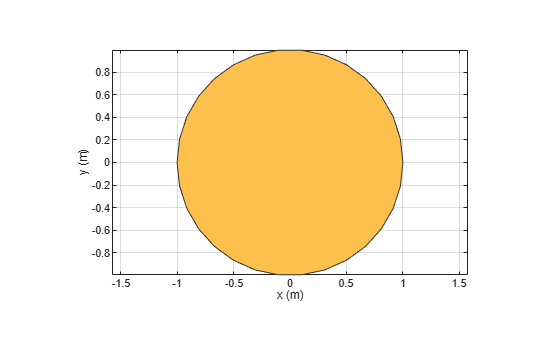```c.NumPoints = 6; c.Radius = 3*ant.Radius; figure show(c)```Create the PCB stack using the vertices derived from the circle shape.

```v = getShapeVertices(c); cp = antenna.Polygon( 'Vertices' ,v); pb = pcbStack(ant); pb.Layers{3} = cp; pb.BoardShape = cp; show(pb) axis equal```Balanis, C. A. Antenna Theory. Analysis and Design. 3rd Ed. Hoboken, NJ: John Wiley & Sons, 2005.

 Stutzman, W. L. and Gary A. Thiele. Antenna Theory and Design. 3rd Ed. River Street, NJ: John Wiley & Sons, 2013.